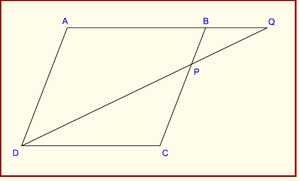### Examination Questions in Parallelograms

###### Click on the option you think is right and then check by clicking on the Show Answer button. You can change your option as many times as you like.

1. Let ABCD be a parallelogram. Let P, Q, R, S be the mid-points of sides AB, BC, CD, DA respectively. Consider the following statements:

1. Area of triangle APS < Area of triangle DSR if BD < AC.
2. Area of triangle ABC = 4 (Area of triangle BPQ).

Select the correct answer using the code given below:

1. 1 only
2. 2 only
3. Both 1 and 2
4. Neither 1 nor 2

2. Consider the following statements:

1. Let ABCD be a parallelogram which is not a rectangle. Then 2(AB 2 + BC 2) ≠ AC 2 + BD 2
2. If ABCD is a rhombus with AB = 4 cm, then AC 2 + BD 2 = n 3 for some positive integer n.

Which of the above statements is/are correct?

1. 1 only
2. 2 only
3. Both 1 and 2
4. Neither 1 nor 2

3. ABCD is a parallelogram. E is a point on BC such that BE : EC = m : n. If AE and DB intersect in F, then what is the ratio of the area of triangle FEB to the area of triangle AFD?

1. m/n
2. (m/n)2
3. (n/m)2
4. [m/(m + n)]2

4. One side of a parallelogram is 8.06 cm and its perpendicular distance from opposite side is 2.08 cm. What is the approximate area of the parallelogram?

1. 12.56 cm2
2. 14.56 cm2
3. 16.76 cm2
4. 22.56 cm2

5. Consider the following statements :

1. If the diagonals of a parallelogram ABCD are perpendicular, then ABCD may be a rhombus.
2. If the diagonals of a quadrilateral ABCD are equal and perpendicular, then ABCD is a square.

Which of the statements given above is/are correct?

1. 1 only
2. 2 only
3. Both 1 and 2
4. Neither 1 nor 2

6. ABCD is a parallelogram. If the bisectors of the angle A and angle C meet the diagonal BD at points P and Q respectively, then which one of the following is correct?

1. PCQA is a straight line
2. Triangle APQ is similar to triangle PCQ
3. AP = CP
4. AP = AQ

7. Let ABCD be a parallelogram. Let m, n be positive integers such that n < m < 2n. Let AC = 2mn and BD = m2 – n2. Let AB = (m2 + n2)/2.

1. Statement –I: AC > BD.
2. Statement- II: ABCD is a rhombus.

Which one of the following is correct in respect of the above statements?

1. Both statement-I and statement-II are true and statement-II is the correct explanation of statement-I
2. Both statement-I and statement-II are true but statement-II is not the correct explanation of statement-I
3. Statement-I is true, but statement-II is false
4. Statement-II is true, but statement-I is false

8. The sides of a parallelogram are 12 cm and 8 cm long and one of the diagonals is 10 cm long. If d is the length of other diagonal, then which one of the following is correct?

1. d < 8 cm
2. 8 cm < d < 10 cm
3. 10 cm < d < 12 cm
4. d > 12 cm

9. Let LMNP be a parallelogram and NR be perpendicular to LP. If the area of the parallelogram is six times the area of triangle RNP and RP = 6 cm, what is LR equal to?

1. 15 cm
2. 12 cm
3. 9 cm
4. 8 cm10. In the figure given above, ABCD is a parallelogram. P is a point on BC such that PB : PC = 1 : 2. DP produced meets AB produced at Q. If the area of the triangle BPQ is 20 square units, what is the area of the triangle DCP?

1. 20 square units
2. 30 square units
3. 40 square units
4. None of the above